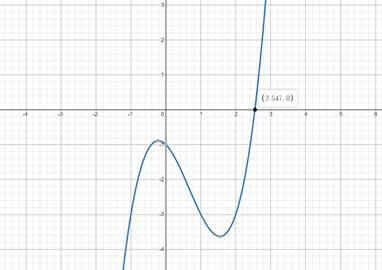# All the real solutions of given equation.### Precalculus: Mathematics for Calcu...

6th Edition
Stewart + 5 others
Publisher: Cengage Learning
ISBN: 9780840068071### Precalculus: Mathematics for Calcu...

6th Edition
Stewart + 5 others
Publisher: Cengage Learning
ISBN: 9780840068071

#### Solutions

Chapter 1.9, Problem 55E
To determine

## All the real solutions of given equation.

Expert Solution

x=2.55

### Explanation of Solution

Given information:

x32x2x1=0

Formula used:

Solving by making graph.

Graphically:

Making Graph of x32x2x1=0 and finding approximate value of x.Graph intersect x-axis at (2.55,0)

x=2.55

### Have a homework question?

Subscribe to bartleby learn! Ask subject matter experts 30 homework questions each month. Plus, you’ll have access to millions of step-by-step textbook answers!